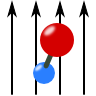# Forces and Movement

In the following problems we will be concerned with the movement of charged particles under the influence of an electrostatic field. Depending on the question we will have to solve Euler-Lagrange equations of motion or use similar approaches. A lot of the problems are historically motivated to which we shall refer in the respective background sections.

## Taming Ions: the Paul TrapIn this problem we want to understand the mechanism behind ion trapping. We will study the movement of charged particles in a so-called Paul trap by solving the equations of motion using approximative methods.

## Geodesic Movement of a Charge on a Surface with Electric FieldThe theoretical description of the movement of a particle in some curved 3D-space and the movement of it on a 2D-surface are equivalent. Both entities are described by a metric and the equation of motion is the geodesic equation. Further giving the particle a charge lets it further interact with an electric field. In this problem you can learn to derive the corresponding geodesic equation.

## The Movement of a Dipolar Molecule in a Constant Electric FieldA lot of molecules can be seen in a first approximation as two charges with a fixed separation. In this problem you will learn what happens if such a molecule is exposed to a constant electric field, especially, what kind of interesting movements one can observe. You will also be able to apply some of your knowledge of mechanics.